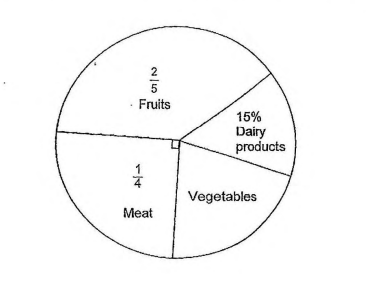# Question 1 of 71

The pie chart below shown the amount of money collected from the sales of different types of items in a supermarket in a week.
(a) if the amount of money collected from the sales of all the items was $50,000 in a week. How much money was collected from the sales of the dairy products? (b)What fraction of the money collected was from the sales of the vegetables?A (a) 6000 (b)$\frac{1}{2}$B (a) 6500 (b)$\frac{1}{3}$C (a) 7000 (b)$\frac{1}{4}$D (a) 7500 (b)$\frac{1}{5}\$
E
None of the above